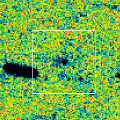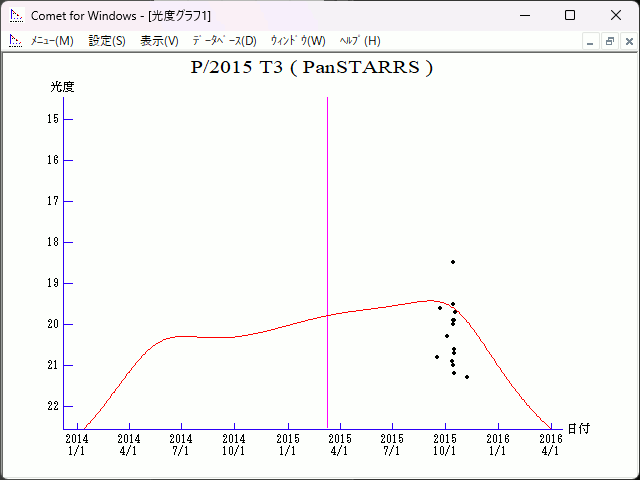# \$B%Q%s%9%?!<%:WB@1(B

466P/PanSTARRS (2015)###\$B%W%m%U%#!<%k(B

 \$BId9f(B 466P/2015 T3 \$BH/8+F|(B 2015\$BG/(B10\$B7n(B13\$BF|(B \$BH/8+8wEY(B 20.9\$BEy(B \$BH/8+ Pan-STARRS 1 telescope (Haleakala)

###\$B###\$B50F;MWAG(B

```                    Epoch = 2015 Feb. 27.0 TT
T = 2015 Mar. 10.15371 TT        Peri. = 196.60342
e = 0.4650167                    Node  = 119.89850 2000.0
q = 2.1694469 AU                 Incl. =  12.22038
a =  4.0551675 AU   n = 0.12069544   P =   8.17 years
```

###\$B8wEYJQ2=(B

```        m1 = 14.0 + 5 log\$B&\$(B + 10.0 log r
```##### \$B50F;MWAG\$O!"(BCBET 5277\$B\$K7G:\\$5\$l\$?\$b\$N\$G\$9!#(B \$B8wEY%0%i%U\$O(BComet for Windows\$B\$G:n@.\$7\$?\$b\$N\$G\$9!#(B